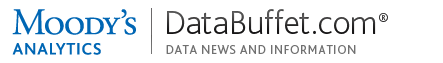Logout / Access Other productsGet live help Monday-Friday from 7:00AM-6:00PM ET (11:00AM-10:00PM GMT)  •  Contact Us
Check out our new FAQ section!
TitleUsing Data Buffet: The CONVERT function
AuthorPhillip Thorne
Question

How can I use the Data Buffet CONVERT function in a basket for precise control when changing the frequency of a time series, or to extract the period-end value?

Data Buffet is a portal to a time series database, which among other functions, permits easy conversion between frequencies (unlike a relational database or spreadsheet). You can convert from the native frequency to a higher frequency (sometimes called "up-converting," which is mathematically disaggregation or  interpolation) or to a lower frequency ("down-converting," which is aggregation and has the effect of smoothing); you can compute the average or sum over a period; or extract either the beginning or end value from a period.

You can use the "Frequency" drop-down control in the Basket module to convert using default settings. For additional flexibility, you will need to write a Data Buffet formula using the CONVERT function.

(Similar drop-down controls are provided by the View, Chart and Map modules, and in the Moody's Analytics Power Tools series wizard. Formulas can be used in all four. Because frequency conversion is a purely mathematical process, the output is not guaranteed to satisfy all topical constraints; please sanity-check before use.)

Syntax

To mark an expression as a formula, enclose it in parentheses. Case and whitespace are ignored. Many of the keywords can be abbreviated. Wild cards and geo lists cannot be used with formulas.

There are two forms of the CONVERT function, with two or four arguments:

(Convert(mnemonic, frequency))
or
(Convert(mnemonic, frequency, technique, observed))

• Mnemonic = the series you're converting.
• Frequency = Annual, A, Quarterly, Q, Monthly, Weekly, etc.
• Technique = Cubic, Constant, Discrete, Linear. For low-to-high conversion, Cubic is usually the best choice, and is Data Buffet's default. For high-to-low conversion, use Discrete.
• Observed = Average, Begin, End, Sum. This overrides the attribute on the series.

The "observed" attribute

Every Data Buffet time series has an "observed" metadata attribute which controls how frequency conversions (i.e., aggregation and disaggregation) apply. It is used by the drop-down controls, and is listed in Mnemonic 411. In general, "stock" measures (balance, inventory, volume, etc.) can be converted as END or AVERAGE, and "flow" measures (transactions) as SUM. When possible, we assign per the source's policy (which we can determine if the same variable is reported at multiple frequencies). Read more about the meaning of the "observed" attribute.

Some series should not be converted; they are statistically valid only at their native frequency. Because we cannot present conversion, we assign an "observed" attribute that produces the "least wrong" answer. For such advisories, please check Mnemonic 411 for written background (for example: the MBA National Delinquency Survey) or a specific FAQ (for example: RealtyTrac foreclosure counts).

Examples of extraction

Extract the last daily value of each month (capitalization is ignored):

(convert(SP500D.IUSA, monthly, discrete, end))

Extract the first daily value of each quarter (the parameters can be abbreviated):

(convert(sp500d.iusa, q, dis, begin))

Examples, high frequency to low

For this direction, to match the output of the "frequency" control on Data Buffet, always use the DISCRETE technique. DISCRETE uses an unweighted average (for example, Q1=(M1+ M2+M3)/3), whereas CUBIC uses a real-time axis and a weighted average (for example, the number of days varies by month).

D»M. Smooth the daily S&P 500 stock price index to a monthly average:

(Convert(SP500D.IUSA, MONTHLY, DISCRETE, AVERAGE))

D»M. Compute the monthly sum of daily trading volume on the NYSE (whitespace is ignored):

(convert(NYGVOLD.IUSA,M,DIS,SUM))

M»A. Smooth a monthly series to an average over the standard January-December calendar year (using the default "observed" attribute of the series):

(CONVERT(LBR.IUSA_MABI, A))

M»A. Smooth the monthly U.S. federal budget deficit over the federal fiscal year, which is October to September:

(Convert(GFB.IUSA, ANNUAL(SEPTEMBER)))

Examples, low frequency to high

For this direction, the CUBIC technique is the default, but you can specify it explicitly in the formula.

Q»M. Convert a quarterly forecast series (based on monthly historical data) to monthly. However, this will not and cannot reconstitute the original irregularity in the monthly historical driver (see related article).

(CONVERT(FLBR.IUSA_MABI, M))

What you can't do

Want to change the observed attribute from AVERAGE to END? This does not work, because the input is already a monthly series:

(convert(SP500AM.IUSA, m, discrete, end))